xReciprocal latticeEncyclopedia
In physics
Physics
Physics is a natural science that involves the study of matter and its motion through spacetime, along with related concepts such as energy and force. More broadly, it is the general analysis of nature, conducted in order to understand how the universe behaves.Physics is one of the oldest academic...

, the reciprocal
Multiplicative inverse
In mathematics, a multiplicative inverse or reciprocal for a number x, denoted by 1/x or x−1, is a number which when multiplied by x yields the multiplicative identity, 1. The multiplicative inverse of a fraction a/b is b/a. For the multiplicative inverse of a real number, divide 1 by the...

lattice
of a lattice (usually a Bravais lattice) is the lattice in which the Fourier transform
Fourier transform
In mathematics, Fourier analysis is a subject area which grew from the study of Fourier series. The subject began with the study of the way general functions may be represented by sums of simpler trigonometric functions...

of the spatial function of the original lattice (or direct lattice) is represented. This space is also known as momentum space or less commonly k-space, due to the relationship between the Pontryagin duals
Pontryagin duality
In mathematics, specifically in harmonic analysis and the theory of topological groups, Pontryagin duality explains the general properties of the Fourier transform on locally compact groups, such as R, the circle or finite cyclic groups.-Introduction:...

momentum and position. The reciprocal lattice of a reciprocal lattice is the original or direct lattice.

## Mathematical description

Consider a set of points R constituting a Bravais lattice, and a plane wave
Plane wave
In the physics of wave propagation, a plane wave is a constant-frequency wave whose wavefronts are infinite parallel planes of constant peak-to-peak amplitude normal to the phase velocity vector....

defined by: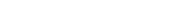If this plane wave has the same periodicity
Periodic function
In mathematics, a periodic function is a function that repeats its values in regular intervals or periods. The most important examples are the trigonometric functions, which repeat over intervals of length 2π radians. Periodic functions are used throughout science to describe oscillations,...

as the Bravais lattice, then it satisfies the equation: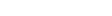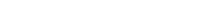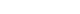Mathematically, we can describe the reciprocal lattice as the set of all vectors K that satisfy the above identity for all lattice point position vectors R. This reciprocal lattice is itself a Bravais lattice, and the reciprocal of the reciprocal lattice is the original lattice, which reveals the Pontryagin duality of their respective vector spaces.

For an infinite three dimensional lattice, defined by its primitive vector
Primitive cell
Used predominantly in geometry, solid state physics, and mineralogy, particularly in describing crystal structure, a primitive cell is a minimum cell corresponding to a single lattice point of a structure with translational symmetry in 2 dimensions, 3 dimensions, or other dimensions...

s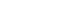, its reciprocal lattice can be determined by generating its three reciprocal primitive vectors, through the formulae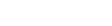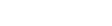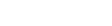Note that the denominator is the scalar triple product. Using column vector representation of (reciprocal) primitive vectors, the formulae above can be rewritten using matrix inversion: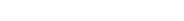This method appeals to the definition, and allows generalization to arbitrary dimensions. The cross product formula dominates introductory materials on crystallography.

The above definition is called the "physics" definition, as the factor of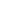comes naturally from the study of periodic structures. An equivalent definition, the "crystallographer's" definition, comes from defining the reciprocal lattice to be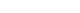which changes the definitions of the reciprocal lattice vectors to be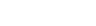and so on for the other vectors. The crystallographer's definition has the advantage that the definition of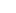is just the reciprocal magnitude of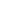in the direction of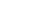, dropping the factor of. This can simplify certain mathematical manipulations, and expresses reciprocal lattice dimensions in units of spatial frequency
Spatial frequency
In mathematics, physics, and engineering, spatial frequency is a characteristic of any structure that is periodic across position in space. The spatial frequency is a measure of how often sinusoidal components of the structure repeat per unit of distance. The SI unit of spatial frequency is...

. It is a matter of taste which definition of the lattice is used, as long as the two are not mixed.

Each point (hkl) in the reciprocal lattice corresponds to a set of lattice planes (hkl) in the real space
Real space
Position space denominates the space of possible locations of an object in classical physics.The real space coordinates specify the position of an object. For instance,...

lattice. The direction of the reciprocal lattice vector corresponds to the normal to the real space planes. The magnitude of the reciprocal lattice vector is given in reciprocal length
Reciprocal length
Reciprocal length or inverse length is a measurement used in several branches of science and mathematics. As the reciprocal of length, common units used for this measurement include the reciprocal metre or inverse metre , the reciprocal centimetre or inverse centimetre , and, in optics, the...

and is equal to the reciprocal of the interplanar spacing of the real space planes.

The reciprocal lattice plays a fundamental role in most analytic studies of periodic structures, particularly in the theory of diffraction
Dynamical theory of diffraction
The dynamical theory of diffraction describes the interaction of waves with a regular lattice. The wave fields traditionally described are X-rays, neutrons or electrons and the regular lattice, atomic crystal structures or nanometer scaled multi-layers or self arranged systems...

. For Bragg reflections
Bragg's law
In physics, Bragg's law gives the angles for coherent and incoherent scattering from a crystal lattice. When X-rays are incident on an atom, they make the electronic cloud move as does any electromagnetic wave...

in neutron
Neutron diffraction
Neutron diffraction or elastic neutron scattering is the application of neutron scattering to the determination of the atomic and/or magnetic structure of a material: A sample to be examined is placed in a beam of thermal or cold neutrons to obtain a diffraction pattern that provides information of...

and X-ray
X-ray
X-radiation is a form of electromagnetic radiation. X-rays have a wavelength in the range of 0.01 to 10 nanometers, corresponding to frequencies in the range 30 petahertz to 30 exahertz and energies in the range 120 eV to 120 keV. They are shorter in wavelength than UV rays and longer than gamma...

diffraction
Diffraction
Diffraction refers to various phenomena which occur when a wave encounters an obstacle. Italian scientist Francesco Maria Grimaldi coined the word "diffraction" and was the first to record accurate observations of the phenomenon in 1665...

, the momentum difference between incoming and diffracted X-rays of a crystal is a reciprocal lattice vector. The diffraction pattern of a crystal can be used to determine the reciprocal vectors of the lattice. Using this process, one can infer the atomic arrangement of a crystal.

The Brillouin zone
Brillouin zone
In mathematics and solid state physics, the first Brillouin zone is a uniquely defined primitive cell in reciprocal space. The boundaries of this cell are given by planes related to points on the reciprocal lattice. It is found by the same method as for the Wigner–Seitz cell in the Bravais lattice...

is a primitive unit cell of the reciprocal lattice.

## Reciprocal lattices of various crystals

Reciprocal lattices for the cubic crystal system are as follows.

### Simple cubic lattice

The simple cubic Bravais lattice, with cubic primitive cell
Primitive cell
Used predominantly in geometry, solid state physics, and mineralogy, particularly in describing crystal structure, a primitive cell is a minimum cell corresponding to a single lattice point of a structure with translational symmetry in 2 dimensions, 3 dimensions, or other dimensions...

of side, has for its reciprocal a simple cubic lattice with a cubic primitive cell of side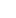(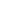in the crystallographer's definition). The cubic lattice is therefore said to be self-dual, having the same symmetry in reciprocal space as in real space.

### Face-centered cubic (FCC) lattice

The reciprocal lattice to an FCC lattice is the body-centered cubic (BCC) lattice.

Consider an FCC compound unit cell. Locate a primitive unit cell of the FCC, i.e., a unit cell with one lattice point. Now take one of the vertices of the primitive unit cell as the origin. Give the basis vectors of the real lattice. Then from the known formulae you can calculate the basis vectors of the reciprocal lattice. These reciprocal lattice vectors of the FCC represent the basis vectors of a BCC real lattice. Note that the basis vectors of a real BCC lattice and the reciprocal lattice of an FCC resemble each other in direction but not in magnitude.

### Body-centered cubic (BCC) lattice

The reciprocal lattice to a BCC
Cubic crystal system
In crystallography, the cubic crystal system is a crystal system where the unit cell is in the shape of a cube. This is one of the most common and simplest shapes found in crystals and minerals....

lattice is the FCC
Cubic crystal system
In crystallography, the cubic crystal system is a crystal system where the unit cell is in the shape of a cube. This is one of the most common and simplest shapes found in crystals and minerals....

lattice.

It can be easily proven that only the Bravais lattices which have 90 degrees between(cubic, tetragonal, orthorhombic) have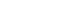parallel to their real-space vectors.

### Simple hexagonal lattice

The reciprocal to a simple hexagonal Bravais lattice with lattice constants c and a is another simple hexagonal lattice with lattice constants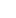and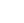rotated through 30° about the c axis with respect to the direct lattice.

## Proof that the reciprocal lattice of the reciprocal lattice is the direct lattice

From its definition we know that the vectors of the Bravais lattice must be closed under vector addition and subtraction. Thus it is sufficient to say that if we have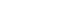and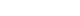then the sum and difference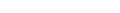satisfy the same.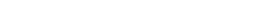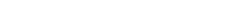Thus we have shown the reciprocal lattice is closed under vector addition and subtraction. Furthermore, we know that a vector K in the reciprocal lattice can be expressed as a linear combination of its primitive vectors.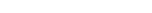From our earlier definition of, we can see that: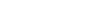where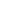is the Kronecker delta. We let R be a vector in the direct lattice, which we can express as a linear combination of its primitive vectors.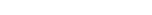From this we can see that: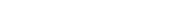From our definition of the reciprocal lattice we have shown that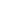must satisfy the following identity.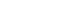For this to hold we must have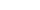equal totimes an integer. This is fulfilled because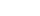and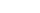. Therefore, the reciprocal lattice is also a Bravais lattice.
Furthermore, if the vectorsconstruct a reciprocal lattice, it is clear that any vector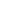satisfying the equation: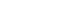...is a reciprocal lattice vector of the reciprocal lattice. Due to the definition of, whenis the direct lattice vector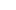, we have the same relationship.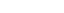And so we can conclude that the reciprocal lattice of the reciprocal lattice is the original direct lattice.

## Arbitrary collection of atoms

One path to the reciprocal lattice of an arbitrary collection of atoms comes from the idea of scattered waves in the Fraunhofer
Fraunhofer diffraction
In optics, the Fraunhofer diffraction equation is used to model the diffraction of waves when the diffraction pattern is viewed at a long distance from the diffracting object, and also when it is viewed at the focal plane of an imaging lens....

(long-distance or lens back-focal-plane) limit as a Huygens-style
Huygens-Fresnel principle
The Huygens–Fresnel principle is a method of analysis applied to problems of wave propagation both in the far-field limit and in near-field diffraction.-History:...

sum of amplitudes from all points of scattering (in this case from each individual atom). This sum is denoted by the complex amplitude F in the equation below, because it is also the Fourier transform
Fourier transform
In mathematics, Fourier analysis is a subject area which grew from the study of Fourier series. The subject began with the study of the way general functions may be represented by sums of simpler trigonometric functions...

(as a function of spatial frequency or reciprocal distance) of an effective scattering potential in direct space: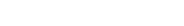Here g = q/(2π) is the scattering vector q in crystallogapher units, N is the number of atoms, fj[g] is the atomic scattering factor for atom j and scattering vector g, while rj is the vector position of atom j. Note that the Fourier phase depends on one's choice of coordinate origin.

For the special case of an infinite periodic crystal, the scattered amplitude F = M Fhkl from M unit cells (as in the cases above) turns out to be non-zero only for integer values of (hkl), where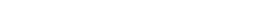when there are j=1,m atoms inside the unit cell whose fractional lattice indices are respectively {uj,vj,wj}. To consider effects due to finite crystal size, of course, a shape convolution for each point or the equation above for a finite lattice must be used instead.

Whether the array of atoms is finite or infinite, one can also imagine an "intensity reciprocal lattice" I[g], which relates to the amplitude lattice F via the usual relation I = F*F where F* is the complex conjugate of F. Since Fourier transformation is reversible, of course, this act of conversion to intensity tosses out "all except 2nd moment" (i.e. the phase) information. For the case of an arbitrary collection of atoms, the intensity reciprocal lattice is therefore: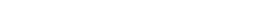Here rjk is the vector separation between atom j and atom k. One can also use this to predict the effect of nano-crystallite shape, and subtle changes in beam orientation, on detected diffraction peaks even if in some directions the cluster is only one atom thick. On the down side, scattering calculations using the reciprocal lattice basically consider an incident plane wave. Thus after a first look at reciprocal lattice (kinematic scattering) effects, beam broadening and multiple scattering (i.e. dynamical
Dynamical theory of diffraction
The dynamical theory of diffraction describes the interaction of waves with a regular lattice. The wave fields traditionally described are X-rays, neutrons or electrons and the regular lattice, atomic crystal structures or nanometer scaled multi-layers or self arranged systems...

) effects may be important to consider as well.

## Generalization of a dual lattice

There are actually two versions in mathematics
Mathematics
Mathematics is the study of quantity, space, structure, and change. Mathematicians seek out patterns and formulate new conjectures. Mathematicians resolve the truth or falsity of conjectures by mathematical proofs, which are arguments sufficient to convince other mathematicians of their validity...

of the abstract dual lattice concept, for a given lattice
Lattice (group)
In mathematics, especially in geometry and group theory, a lattice in Rn is a discrete subgroup of Rn which spans the real vector space Rn. Every lattice in Rn can be generated from a basis for the vector space by forming all linear combinations with integer coefficients...

L in a real vector space
Vector space
A vector space is a mathematical structure formed by a collection of vectors: objects that may be added together and multiplied by numbers, called scalars in this context. Scalars are often taken to be real numbers, but one may also consider vector spaces with scalar multiplication by complex...

V, of finite dimension.

The first, which generalises directly the reciprocal lattice construction, uses Fourier analysis. It may be stated simply in terms of Pontryagin duality
Pontryagin duality
In mathematics, specifically in harmonic analysis and the theory of topological groups, Pontryagin duality explains the general properties of the Fourier transform on locally compact groups, such as R, the circle or finite cyclic groups.-Introduction:...

. The dual group
Dual group
In mathematics, the dual group may be:* The Pontryagin dual of a locally compact abelian group* The Langlands dual of a reductive algebraic group* The Deligne-Lusztig dual of a reductive group over a finite field....

V^ to V is again a real vector space, and its closed subgroup L^ dual to L turns out to be a lattice in V^. Therefore L^ is the natural candidate for dual lattice, in a different vector space (of the same dimension).

The other aspect is seen in the presence of a quadratic form
In mathematics, a quadratic form is a homogeneous polynomial of degree two in a number of variables. For example,4x^2 + 2xy - 3y^2\,\!is a quadratic form in the variables x and y....

Q on V; if it is non-degenerate it allows an identification of the dual space
Dual space
In mathematics, any vector space, V, has a corresponding dual vector space consisting of all linear functionals on V. Dual vector spaces defined on finite-dimensional vector spaces can be used for defining tensors which are studied in tensor algebra...

V* of V with V. The relation of V* to V is not intrinsic; it depends on a choice of Haar measure
Haar measure
In mathematical analysis, the Haar measure is a way to assign an "invariant volume" to subsets of locally compact topological groups and subsequently define an integral for functions on those groups....

(volume element) on V. But given an identification of the two, which is in any case well-defined
Well-defined
In mathematics, well-definition is a mathematical or logical definition of a certain concept or object which uses a set of base axioms in an entirely unambiguous way and satisfies the properties it is required to satisfy. Usually definitions are stated unambiguously, and it is clear they satisfy...

up to a scalar
Scalar (mathematics)
In linear algebra, real numbers are called scalars and relate to vectors in a vector space through the operation of scalar multiplication, in which a vector can be multiplied by a number to produce another vector....

, the presence of Q allows one to speak to the dual lattice to L while staying within V.

In mathematics
Mathematics
Mathematics is the study of quantity, space, structure, and change. Mathematicians seek out patterns and formulate new conjectures. Mathematicians resolve the truth or falsity of conjectures by mathematical proofs, which are arguments sufficient to convince other mathematicians of their validity...

, the dual lattice of a given lattice
Lattice (discrete subgroup)
In Lie theory and related areas of mathematics, a lattice in a locally compact topological group is a discrete subgroup with the property that the quotient space has finite invariant measure...

L in an abelian
Abelian group
In abstract algebra, an abelian group, also called a commutative group, is a group in which the result of applying the group operation to two group elements does not depend on their order . Abelian groups generalize the arithmetic of addition of integers...

locally compact
Locally compact space
In topology and related branches of mathematics, a topological space is called locally compact if, roughly speaking, each small portion of the space looks like a small portion of a compact space.-Formal definition:...

topological group
Topological group
In mathematics, a topological group is a group G together with a topology on G such that the group's binary operation and the group's inverse function are continuous functions with respect to the topology. A topological group is a mathematical object with both an algebraic structure and a...

G is the subgroup L of the dual group
Dual group
In mathematics, the dual group may be:* The Pontryagin dual of a locally compact abelian group* The Langlands dual of a reductive algebraic group* The Deligne-Lusztig dual of a reductive group over a finite field....

of G consisting of all continuous characters that are equal to one at each point of L.
In discrete mathematics, a lattice is a locally discrete set of points described by all integral linear combinations of dim = n linearly independent vectors in Rn. The dual lattice is then defined by all points in the linear span of the original lattice (typically all of R^n) with the property that an integer results from the inner product with all elements of the original lattice. It follows that the dual of the dual lattice is the original lattice.
Furthermore, if we allow the matrix B to have columns as the linearly independent vectors that describe the lattice, then the matrix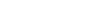has columns of vectors that describe the dual lattice.

## Reciprocal space

Reciprocal space (also called "k-space") is the space in which the Fourier transform
Fourier transform
In mathematics, Fourier analysis is a subject area which grew from the study of Fourier series. The subject began with the study of the way general functions may be represented by sums of simpler trigonometric functions...

of a spatial function is represented (similarly the frequency domain
Frequency domain
In electronics, control systems engineering, and statistics, frequency domain is a term used to describe the domain for analysis of mathematical functions or signals with respect to frequency, rather than time....

is the space in which the Fourier transform of a time dependent function is represented). A Fourier transform takes us from "real space" to reciprocal space or vice versa.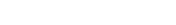A reciprocal lattice is a periodic set of points in this space, and contains the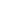points that compose the Fourier transform of a periodic spatial lattice. The Brillouin zone
Brillouin zone
In mathematics and solid state physics, the first Brillouin zone is a uniquely defined primitive cell in reciprocal space. The boundaries of this cell are given by planes related to points on the reciprocal lattice. It is found by the same method as for the Wigner–Seitz cell in the Bravais lattice...

is a volume within this space that contain all the unique k-vectors that represent the periodicity of classical or quantum waves allowed in a periodic structure.